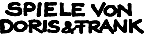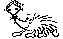Great German Games with a HedgehogOur Games About us Online Games Events Other Contact Order Infos Home  >  Online Games  >  Numfield  >  Do Your Strategy# Numfield, how to Java

Warning, this page is a bit longer than the other ones. You should probably skip this page, if you are not fluent in a computer language similar to C, C++ or of course Java! This page contains of the following chapters:

### The Basic Class

The basic system I've created, works as a Java-applet. The applet demonstrated earlier has kind of a plug-in structure, allowing to easily add any strategies as soon as the are derived of the following class:
 ```abstract class Strategy { int maxSteps; int stepBonus; int opponents; int maxPlayableNumber; // You have to keep that method since it is final final public void init(int maxSteps, int maxPlayableNumber, int stepBonus, int opponents) { this.maxSteps = maxSteps; this.stepBonus = stepBonus; this.opponents = opponents; this.maxPlayableNumber = maxPlayableNumber; priv_init(); } // Overload this procedure to do your own // initialization: void priv_init() { } // Overload this procedure to decide your first turn: abstract public int initial_turn(); // Overload this procedure to decide further turns: abstract public int turn(int last_turns[], int scores[], int carryOver, int turn_no); // This hook is usually ignoreable but was necessary // for the PlayYourSelf strategy! public void destroy() { } public void show() { } public void hide() { } } } ```

Yes I see the coding is not entirely consistent about the naming convention for variables, but I didn't know the Sun conventions when I started that project.

To derive your own version you need to override the following methods:

 priv_init for special initialization initial_turn for the first turn in the game turn for all further turns
Note that you don't have to override priv_init if you don't need it. The other two methods have to be overriden!

The only method of the above which uses parameters is turn . The parameters have the following meaning:

 last_turns contains the last bets of all strategies. Note the strategies own last turn is located at index 0. The existing indices are 0 to (number of players-1). scores contains the accumulated points of all strategies. Indices work as for last_turns. carryOver contains the points of the pot carried over from last round turn_no is the number of the current round.

Remark: Note that the arrays scores and last_turns are consistent to each other and over time. That means for a certain fixed index i0 the particular score scores[i0] is the score of the strategy which bet a last_turns[i0] in the last round. And at the next turn the data at index i0 corresponds to the same strategy!

To make programming easier every sub class of strategy should use the following variables which are set appropriately during initialization:

 maxPlayableNumber The highest number a strategy can play legaly (default is 99). stepBonus amount added to the pot by the bank every turn (default is 1). opponents Number of opponents found during initialization. To find the currently living you have to count dead ones (score less or equal zero). maxSteps has no meaning yet.

If your strategy needs any other information it could derive them from that information and should do its own bookkeeping. Somewhere below there is an example of simple bookkeepingNote: If I get classes which exhaust CPU time or memory I might have to exclude them from participation. But I think there should be many interesting strategies with a reasonable amount of resource consumption.

### A Few Simple Examples

This very simple strategy shows that a simple strategy is coded fairly simple:

 ```class Strat23 extends Strategy { public int initial_turn() { return 2; } public int turn(int last_turns[], int scores[], int carryOver, int turn_no) { // What did we do last time? return last_turns == 2 ? 3 : 2; } } ```

You can also derive your own subclasses which have a common behaviour, e.g.:

 ```class StratConst extends Strategy { public int initial_turn() { return 1; } public int turn(int last_turns[], int scores[], int carryOver, int turn_no) { return last_turns; } } class Const2 extends StratConst { public int initial_turn() { return 2; } } ```

A randomized player:

 ```class Random11 extends Strategy { public int initial_turn() { return (int) (Math.random() * 11.0 + 1); } public int turn(int last_turns[], int scores[], int carryOver, int turn_no) { return initial_turn(); } } ```

This is a very simple strategy which does a bit of own bookkeeping:

 ```class Nihilist extends Strategy { public int average, sum; public int initial_turn() { return 0; } public int turn(int last_turns[], int scores[], int carryOver, int turn_no) { sum += carryOver; average = sum / turn_no; if ( carryOver > average ) return 1; else return 0; } } ```### Two More Complex Strategies

Since a few people asked questions about certain technical problems and how to realize it in Java, here is a slightly more involved strategy, which demonstrates the use of priv_init() to allocate storage and a (inefficient, I know) method to score a turn. First consider this little guy:
 ```// Play the number which would have made highest // gain in last turn. class Shark5 extends Strategy { public int initial_turn() { return 1; } // A tiny little turn-evaluator: int eval(int turns[], int carry, int scores[]) { int i, j, sum, t, parts, t0; sum = carry; parts = 0; t0 = 0; for (i = 0; i < opponents; i++) if ( scores[i] > 0 ) { if ( turns[i] > 0 ) { sum += turns[i]; t = 0; for (j = 0; j < opponents; j++) { if ( turns[j] > 0 && turns[i] >= turns[j] ) t++; } if (i == 0) t0 = t; parts += t; } } sum += stepBonus; if ( parts == 0 ) return 0; return (int) ( sum * t0 ) / parts - turns; } public int turn(int last_turns[], int scores[], int carryOver, int turn_no) { int i, i0, w, w0; w0 = - maxPlayableNumber; i0 = 0; for (i = 0; i <= maxPlayableNumber; i++) { last_turns = i; w = eval(last_turns, carryOver, scores); if ( w > w0 ) { w0 = w; i0 = i; } } return i0; } } ```
Remark: The eval method contained in the above example is not optimized for effience, it is kind of a very first way to do it. Numfield itself uses a much faster version internally. A nice packed version in a separate class byDon Reble is on this appendix page . Anyone interested in clean Java should go there. Note the following strategy is derived from the previous and uses the method eval of the previous one.
 ```// Play the number which would have made highest // gain in last 2 turns. class Shark6 extends Shark5 { public int initial_turn() { return 0; } int lt[]; void priv_init() { // Allocate memory to remember previous turns: lt = new int[opponents]; } public int turn(int last_turns[], int scores[], int carryOver, int turn_no) { int i, i0, w, w0; if ( turn_no <= 1 ) { i0 = 0; } else { w0 = - maxPlayableNumber; i0 = 0; for (i = 0; i <= maxPlayableNumber; i++) { last_turns = i; lt = i; w = eval(last_turns, carryOver, scores) +eval(lt,carryOver, scores); if ( w > w0 ) { w0 = w; i0 = i; } } } for (i = 0; i < opponents; i++) lt[i] = last_turns[i]; return i0; } } ```
The strategy Shark7 in the example run is also derived from Shark5 and a younger (and more successful) brother of shark6.

A few pages further ahead there can be found more samples of strategy source code .

And please forget about the examples: Try s.th. completely different! Just take the examples as demonstrations of the technique.### A Tiny Test Environment For You

Due to repeated demand:
1. To develop and test your own classes you need a Java-Compiler, which should be arround 1000 times at the net. But the best place isSUN-Microsystems . There you find the JDKs: Java Development Kits. Since Numfield is stone age Java any version published should be enough (1.02 and above).
2. Further you should download this zipfile . Note that this File is updated whenever I receive new strategies. But some versions prior 13th of June 1999 either contained incomplete versions of my sample strategies or a broken version of the simulation applet itself. The correct version should claim to be 1.55! I appologize for the mess I might have created...
3. Unpack it to an empty directory.
4. Now you need an applet viewer: You current browser should be enough, if it displayed the numfield applet earlier. JDKs contain a standalone applet viewer, named appletviewer (it is so easy) or applet in older JDKs.
5. Point your viewer to either of the files sentin1.html   or sentin2.html . If its a JDK one you can only see the applet, in a browser you will see a ugly page arround the applet, since I didn't put any pictures in the archive... If you can see the applet running all is fine.
6. As soon as you get the applet running, you need to do the following to do your own programming:
1. Create your strategies .java file.
2. Compile and debug until it runs!
3. If you don't like the Java debugger, use System.out.println(...) statements and watch the output in the Java console.
4. Put its class file (or files for real hackers) in the numfield directory.
5. Put the name of the (main) class of your strategy in a separate line in either of the files sentin1.html or sentin2.html (Look and you will find the right position, I'm sure). Note this is case sensitive!
6. Start your viewer and see your strategy competing!
7. Improve it!
7. Send it to Frank!

OK, thats all by now. Now its your turn!(

http://doris-frank.de/numjava.html changed 4/16/07, rendered 4/17/07
(c) 1997-2007 by   Doris & Frank    Site  imprint